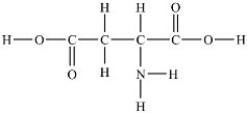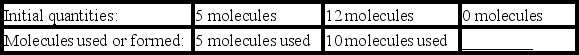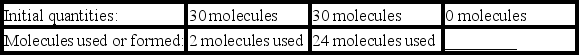# General Organic and Biological Chemistry Study Set 1

Chemistry

## Quiz 5 :Chemical ReactionsLooking for Organic Chemistry Homework Help?

## Quiz 5 :Chemical Reactions

Showing 1 - 20 of 98The law of conservation of mass states that ________.
Free
Multiple Choice

AIdentify the correct classification for the reaction 2 PbSO4 → 2 PbSO3 + O2
Free
Multiple Choice

BIdentify the correct classification for the reaction H2O + SO3 → H2SO4
Free
Multiple Choice

AWhich reaction is a double displacement reaction?
Multiple ChoiceIdentify the correct product that would complete the following double displacement reaction: NaOH + HBr → ___ + H2O
Multiple ChoiceThe Greek letter delta (Δ)may be written over the reaction arrow in a chemical equation to indicate that ________.
Multiple ChoiceWhich chemical equation is properly balanced?
Multiple ChoiceIn the chemical equation 2 Co(NO3)3 + 3 (NH4)2S → Co2S3 + 6 NH4NO3 ,how many nitrogen atoms are on each side of the equation?
Multiple ChoiceWrite a balanced chemical equation for the reaction of acetone (C3H6O)with oxygen (O2)to form carbon dioxide (CO2)and water (H2O).
Multiple ChoiceHow many carbon atoms are in 3.85 mol of carbon?
Multiple ChoiceHow many iron atoms are in 0.32 mol of Fe2O3?
Multiple ChoiceHow many moles of chloroethylene (C2H3Cl)contain 5.47 × 1026 molecules?
Multiple ChoiceWhat is the formula weight of KCl?
Multiple ChoiceWhat is the formula weight of Co(NO3)3?
Multiple ChoiceWhat is the mass of 3.81 mol of PH3?
Multiple ChoiceHow many moles of carbon dioxide are in 211 g of carbon dioxide?
Multiple ChoiceAspartic acid is an amino acid used to synthesize proteins. What is the molar mass of aspartic acid shown below?Multiple ChoiceWhich sample contains the largest number of molecules?
Multiple ChoiceComplete the following table using the given balanced equation and the initial quantities of reactants. Equation: N2(g)+ 2 O2(g)→ 2 NO2(g)Complete the following table using the given balanced equation and the initial quantities of reactants. Equation: S8 + 12 O2 → 8 SO3